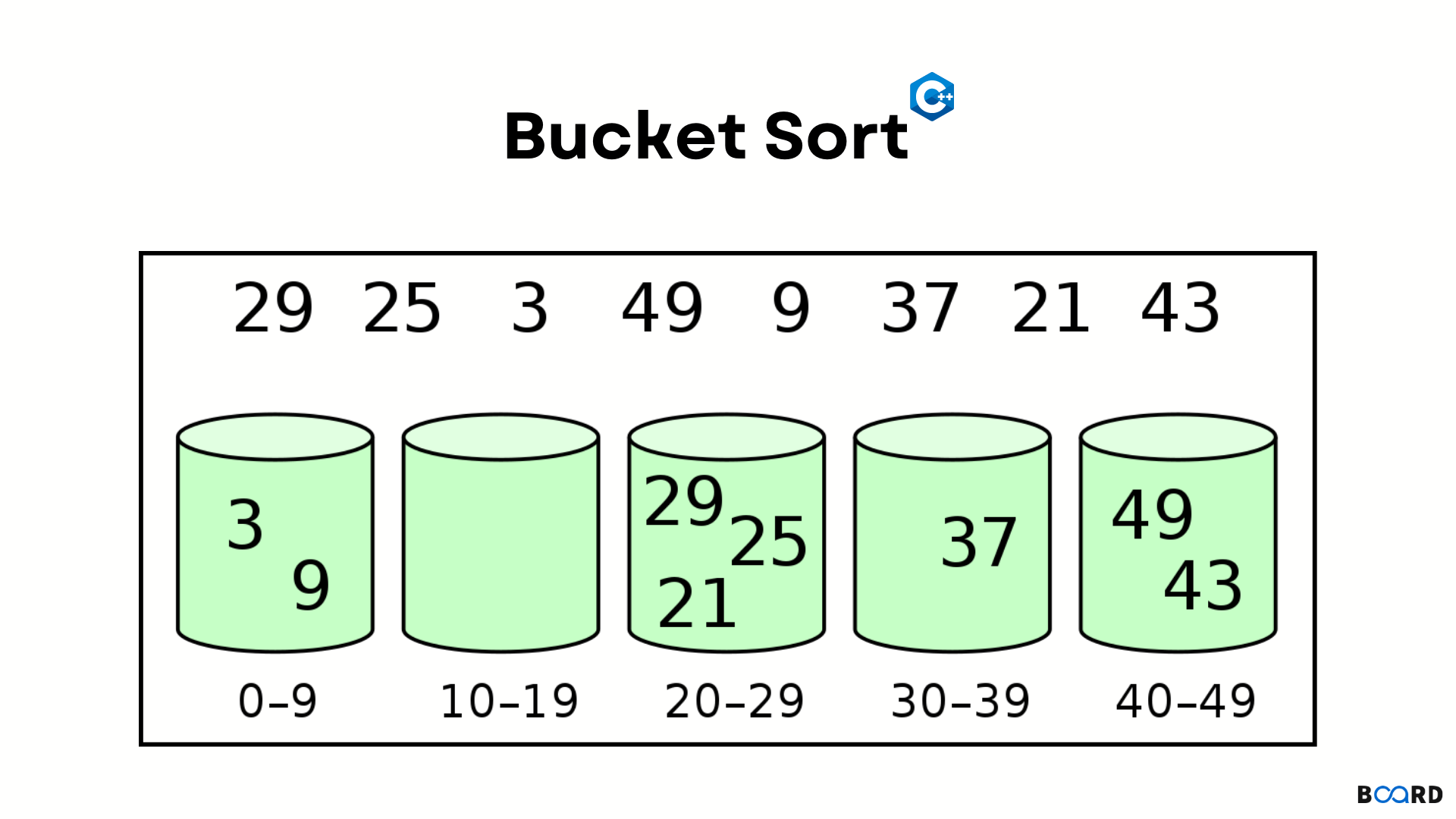# Bucket Sort Algorithm with C++## Overview

Bucket sort is a sorting algorithm that is used mainly when the input is distributed uniformly over a range. Foran instance, sorting a collection of floating point numbers uniformly distributed within the range [0.0, 1.0].

We may use any of the various sorting algorithms available such as quick sort, counting sort, insert sort, etc, each of which operates with varying space/time complexities. When, why, and how to use bucket sort, is something that we learn in this article.

## Scope

• Pseudo-code of the bucket-sort algorithm.
• Explanation and Time complexity for the bucket-sort algorithm.
• Example with Implementation.

## Pseudocode

 bucketSort(arr){    sz = arr.size    create sz empty buckets    do following for every arr[i]:        insert arr[i] into the bucket[n * array[i]]    sort each bucket individually using the insertion sort    concatenate all the sorted buckets.}

## Explanation and Time Complexity

Suppose that inserting in a bucket consumes O(1) time. Thus the first 2 steps would consume O(n) time. The O(1) becomes possible if using a linked list for representing a bucket. The 3rd step also takes an average O(n) time, provided all the numbers are distributed uniformly. The final step also consumes O(n) time as the number of items in all the buckets will be n.

## Implementation

 #include "iostream"#include "vector"#include "algorithm"using namespace std;void bucketSort(vector&arr){    int n = arr.size();    vector> b(n); for (int i = 0; i < n; ++i) { b[n * arr[i]].push_back(arr[i]); } for (auto&bucket : b){ sort(begin(bucket), end(bucket));    } for (int ind=0, i = 0; i < n; ++i){ for (int j = 0; j < b[i].size(); ++j){ arr[ind++] = b[i][j];        }    }}int main(){    vectorv={ 0.3465, 0.632, 0.667, 0.565, 0.1253, 0.667, 0.888 }; bucketSort(v); cout << "The array after sorting is:"<

Output

 The array after sorting is :0.1253 0.3465 0.565 0.632 0.667 0.667 0.888
write your code here: Coding Playground